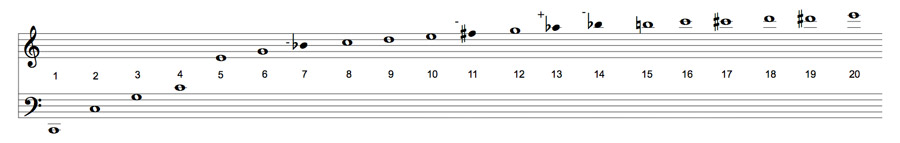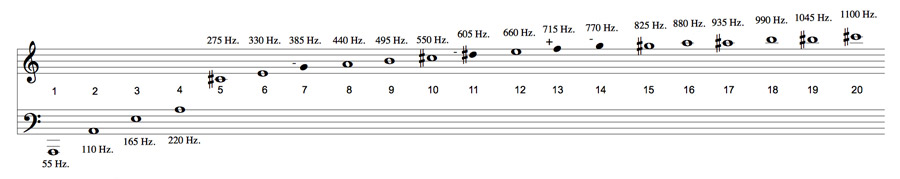A# harmonic seriesFigure 1. The first 20 partials of a harmonic series starting from the fundamental C2 (about 65.4 Hz). Frequency multiples are shown between the staves. The - and + symbols indicate that the notate pitch is significantly lower or higher than the same pitch o a modern piano.Figure 2. The first 20 partials of a harmonic series starting from the fundamental C2 (about 65.4 Hz). Frequency multiples are shown between the staves. The - and + symbols indicate that the notate pitch is significantly lower or higher than the same pitch o a modern piano.

A harmonic series is a series of frequencies the lowest of which is called the fundamental and in which there is a constant difference in frequency between adjacent members. For example, 1,000, 2,000, 3,000, 4,000, ... Hz and 500, 1,500, 2,000, 2,500, ... Hz are both examples of harmonic series, with fundamentals of 1,000 and 500 Hz, respectively. The components higher than the fundamental are known as overtones, harmonics, or partials. The = and - symbols indicate that the notated pitch is significantly lower or higher.

A harmonic series can be expressed in a compact way as follows:

f0, 2f0, 3f0, 4f0, ... (1)

where f0 is the fundamental frequency.

This traditional way of notating the harmonic series is less precise at conveying frequency information than the frequency-multiple notation shown above in (1). However, it does provide a convenient way for musicians to memorize the harmonic series as a kind of scale. Once memorized it may easily be transposed to any fundamental pitch. For example, Figure 2 shows a similar diagram for the first 20 partials of a harmonic series starting from A1 (approx. 55 Hz.).

Here, the frequency of each partial has been also been specified. (The frequency of each partial may be calculated by multiplying the fundamental frequency, in this case 55 Hz, by the partial number indicated between the staves.)